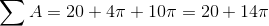## Example Questions

### Example Question #643 : Geometry

The surface area of a cylinder is given by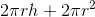, whereis the radius and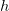is the height.  If a cylinder has a surface area of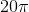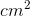and a height of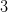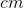, what is its radius?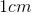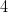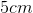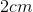Explanation:

The fastest way to solve this problem is to plug all of the answer choices in forand look for an output of.

Alternatively, one could set the surface area formula equal to(knowing that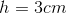), but this would require solving a quadratic.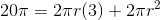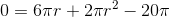Rearranging this we get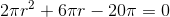Now factoring out awe get: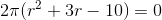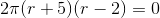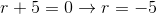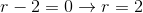Since we cannot have a negative length our answer is.

### Example Question #644 : Geometry

If a half cylinder with a height of 5 and semicircular bases with a radius of 2, what is the surface area?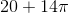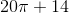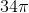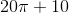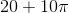Explanation:

The surface area of the half cylinder will consist of the lateral area and the base area.

There are three parts to the surface area:

Rectangular region, semi-circular bases of the half cylinder, and outer face of the cylinder.

The cross section of the half cylinder is a rectangle.  Find the area of the rectangle.  The diameter of the semicircle represents the width of the rectangle, which is double the radius.  The length of the rectangle is the height of the cylinder.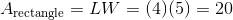Next, find the area of a semicircular bases.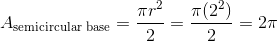Since there are two semicircular bases of the semi-cylinder, the total area of the semicircular bases is.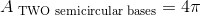Find the area of the outer region.   The area of the outer region is the half circumference multiplied by the height of the cylinder.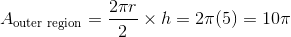Sum the areas of the rectangle, the two circular bases, and the outer region to find the lateral area.